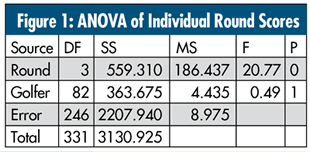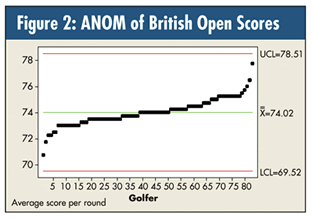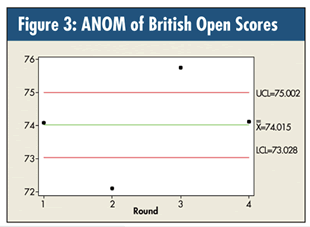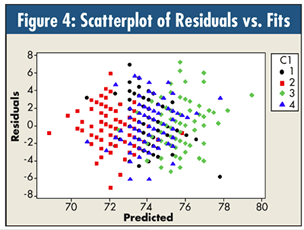# Quality DigestBio

## Considering Golf… Statistically

### A better way to teach “the basics”?

This column is in honor of the first anniversary of my late father’s death. In his last days, Dad enjoyed watching golf, and I’d often join him. Watching the recent British Open, I thought I would apply some basic statistical principles to the final scores.

For example, 83 people made the cut, and the ANOVA of their individual round scores is shown in figure 1.The two ANOM plots are shown in figures 2 and 3.Another interesting statistic is the standard deviation of an individual round: square root 8.975 ~ 3. Using the standard Bartlett and Levene tests for equality of variances, I tested the 83 golfers as to whether this was consistent for all of them:

p-value Bartlett: 0.891

p-value Levene: 0.983

Depending on luck and other random factors, an individual’s score could swing by ± 6-9 strokes in a round!

I also wondered whether the rounds with average higher scores had more variation. So, in figure 4 I did the standard residual vs. predicted value plot and coded it by round (Bartlett p-value: 0.303; Levene p-value: 0.221). The assumption of equal variance seems quite sound.Let’s teach some more basics. Given that the standard deviation of a single round is ~3, then the standard deviation of each individual’s four-round sum is 3 × square root 4 ~ 6. So, if the p-value for the golfer was significant (it wasn’t), the least significant difference (LSD) between two golfers’ summed scores would be: 2 × square root 2 × 6 ~ 17. (The scores ranged from 283 to 311.)

Because the p-value was not significant, one is now forced to use the Studentized range factor for making 83 simultaneous comparisons, which is (best case) ~5.7 × 6 = 34. So, once again: no difference.

From this analysis, it seems possible in any given tournament for someone to have everything go right all four rounds and win a championship by coming in 12-18 strokes under par (2-3 standard deviations on a four-round score) due merely to good luck!

Figures 5 and 6 show a normal plot of the final four-round summed scores and a box-and-whisker plot, respectively.The p-value for normality is < 0.05, and the boxplot finds some outliers (< 288 and > 304).

Over time, one could look at a group of golfers, their rankings in the various high-stakes tournaments, and use ANOVA on the ranks to determine whether someone is consistently a better golfer. I would probably put my money on Tiger Woods.

Well, I’ve had some fun and I hope you did, too… and Dad, I still miss you!

### Discuss